# ------ Draw Shapes ------

We discussed the movement of the turtle. Now, we learn to move on to making actual shape. First, we draw the polygon since they all consist of straight lines connected at the certain angles. So, let start.

# ------ Square ------

Code -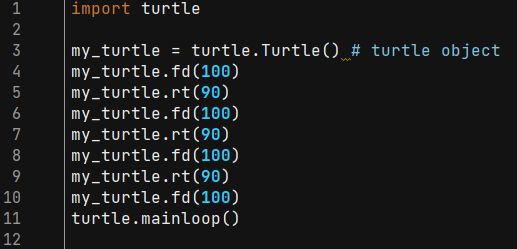Output -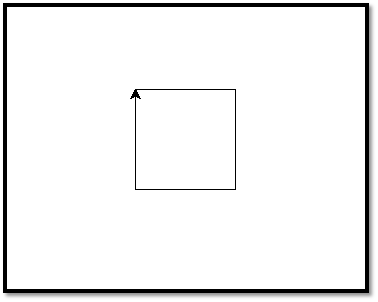# ------ Polygon ------

Code -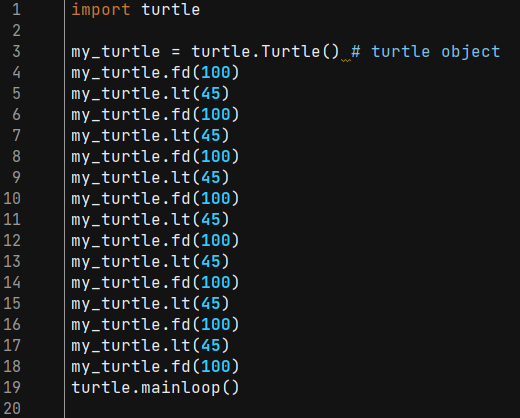Output -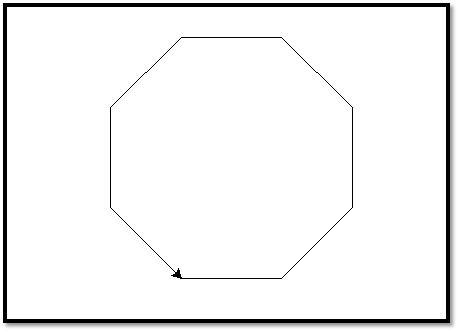Note - We can draw any shape using the turtle, such as a rectangle, triangle, square, and many more. But, we need to take care of coordinate while drawing the rectangle because all four sides are not equal. Once we draw the rectangle, we can even try creating other polygons by increasing number of side.

# ------ Circle ------

circle( radius, extent = None, steps = integer ) :

It is used to draw the circle to the screen. It takes three arguments.

radius - It can be a number.

extent - It can be a number or None.

steps - It can be an integer.

The circle is drawn with the given radius. The extent determines which part of circle is drawn and if the extent is not provided or none, then draw the entire circle. Let's understand the following example.

Code -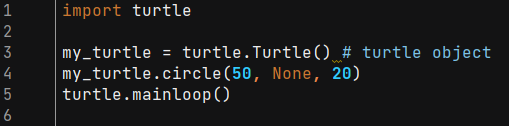Output -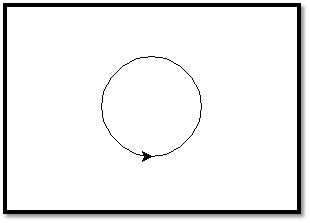# ------ Dot ------

We can also draw a dot, which is also known as a filled-in circle. Follow the given method to draw a filled-in circle.

Code -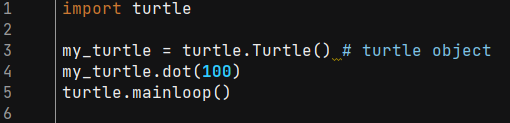Output -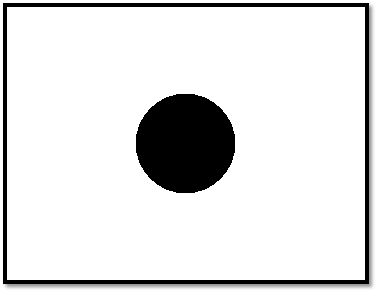Next Article -